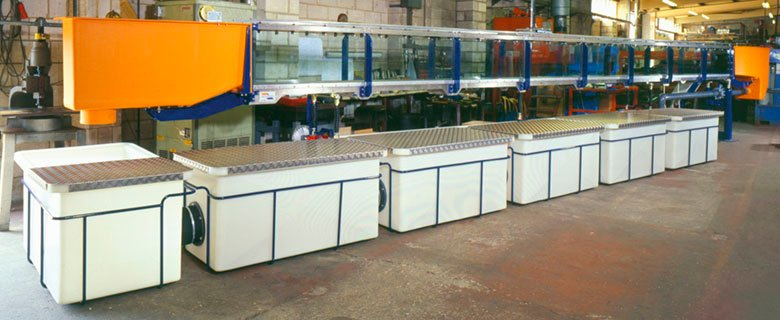# Experiment # 2

## To Investigate the relationship between specific energy (E) and depth of flow (y) in a rectangular channel.

Objectives1. To study the variation in specific energy as a function of depth of flow for a given discharge in a lab flume.
2. To validate the theories of E ~ y diagram.

Apparatus

1. S-6 Tilting laboratory flume with manometer, slope adjusting scale and flow arrangement.
2. Hook gauge (to measure depth of water)Related Theory

## Flume

It is a channel supported above the ground level.

## Specific Energy

It is the total energy per unit weight measured relative to the channel bed. E = y+(V^2)/2g## Assumptions

1. Normal flow conditions exit in channel. ( Steady uniform flow )
2. Velocity correction factor ( α = 1 )
3. Bed Slope is very small. ( Practically S < 1: 10 )## Critical Depth

It is the depth of flow in the channel at which specific energy is minimum## Froud No

It is the ratio of inertial forces to gravitational forces.## Critical Flow

It is the flow corresponding to critical depth. (Fr = 1)

## Sub-Critical Flow

It is the flow with larger depths & less flow velocities. (Fr < 1)

## Super-Critical Flow

It is the flow with lesser depths & larger flow velocities. (Fr > 1)## Emin (Minimum Specific Energy)

It is the specific energy at critical depth under critical velocity condition in the channel## Critical Velocity

Velocity of flow at critical depth is called Critical Velocity.

## Alternate Depths

For any value of Specific energy other then at the critical point for a constant discharge, there are two water depths, one is greater then the critical depth and the other is less then the critical depth. These two depths for a given specific energy are termed as specific depths.## Procedure

1) Maintain constant discharge in the open channel.
2) For one particular value of slope find out the water depths at three different locations and calculate the average depth of flow .
3) Calculate the specific energy using relationship.4) Repeat this procedure for different values of slope.
5) Draw the specific energy curve.6) Find out the critical depth and Emin
7) Find out yc theoretical and Emin theoretical.## Precautions

1) Tip of the hook gauge should just touch the water.